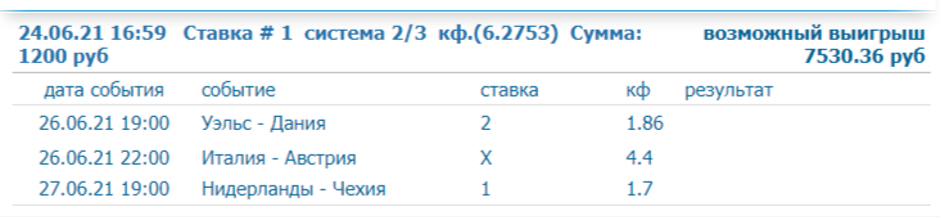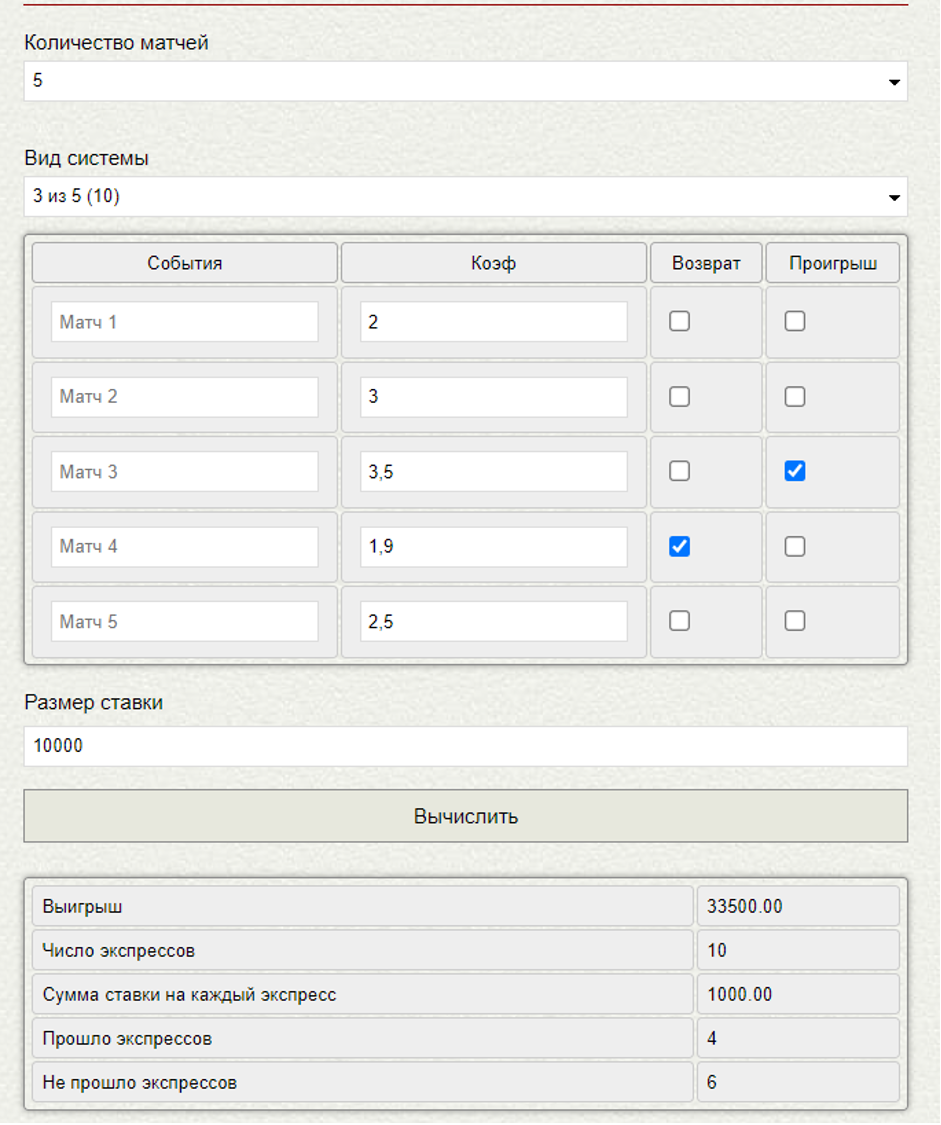# How is the sports betting system calculated?

03.07.2021

What is the accumulator system in sports betting? What a system of express bets can be in a bookmaker, how bookmakers calculate bets on express systems, what odds for an event are better to choose in them. Everything you need to know about this type of bet in our today's material.

## What is the system?

In the classical sense of the word, the system is a system of express trains. Doubles (multiples of two outcomes), triplets (multiples of three outcomes), fours (multiples of four outcomes), and so on, concluded within one bet. To bet on the system, you need to choose at least three outcomes, such a system is called 2/3 or “two out of three”.

***

Express systems always have two numbers. The second of them is the number of selected event outcomes in the system, that is, the player's bets on the event. The first number is the number of selections that must pass to get at least some kind of win.

***

In this way:

1. System "two out of three" - three outcomes of the event, of which at least two must pass;
2. System "five out of six" - six outcomes of the event, of which at least five must pass;
3. System "three out of five" - ​​five outcomes of the event, of which at least three must pass.

The "two out of three" system is the simplest, it is easy to calculate it even in your head or using a simple calculator. But with a larger number of outcomes, it is already much more difficult to calculate the system, and here it is more appropriate to use special calculators to calculate the systems. They can be found, for example, on the websites of many bookmakers.

## How the express system is calculated?

Unlike express and single bets, the system's winnings are not fixed. The player can get different winnings depending on how many outcomes he has entered in the system. It all depends on the number of options that came in. So, for example, in the "four out of six" system, the following options are possible:

1. Three or fewer outcomes of the event won - the bet is lost;
2. Four won outcomes of the event - the minimum winning;
3. Five won outcomes of the event - the winnings increase;
4. Six won outcomes of the event - the maximum possible payout.

Moreover, in the system there are possible variations of the winnings depending on which outcomes of the event and combinations will play. Usually, when betting on the system, the coupon indicates the maximum winnings - how much the player will receive if all his bets pass. But, as we can see, other options are also possible there.

## Express system: calculation example

For simplicity of the example, we will take the simplest system, "two out of three".In total, we put 1,200 rubles on the system. This money was evenly (400 rubles) divided into three doubles. These are the following combinations:

1. Express A: Denmark and Italy draw (odds 8.184);
2. Express B: Denmark and the Netherlands (odds 3.162);
3. Express C: Draw Italy and the Netherlands (odds 7.48).

These coefficients arose from the multiplication of the quotes of the corresponding outcomes.

So, we have three events and three outcomes, which corresponds to the second number in the name of the express system. Remember what the first number stands for? The number of outcomes to play. If all three bets are played, the bookmakers will pay the maximum winnings for the entire bet specified in the coupon - 7,530 rubles and 36 kopecks.

If two bets are played, the winnings will be calculated depending on the past outcomes of the event. If double A passes (Denmark wins and Italy draws), the player will receive 3,273 rubles and 60 kopecks (8.184 x 400). If double B passes (victory of Denmark and the Netherlands), the player will receive the minimum amount of 1,264 rubles and 80 kopecks (3.162 x 400). If double C plays (a draw for Italy and a victory for the Netherlands), then the player will receive 2,992 rubles (7.48 x 400) for this event.

If only one of these outcomes or no event passes, the whole system burns out. Bookmakers are in the black, but the player gets nothing. Such a variety of calculation options is possible even in the simplest "two out of three" system.

Any express system of this type is formed according to the same principle, but the larger the system, the more combinations of calculation it contains.

In the "three out of five" system, you need to choose five outcomes, tees will be created for all possible combinations, and there are already ten of them. To get the minimum win, you need to play at least one of the tees, that is, three bets out of five.

In the "four out of eight" system, there are already eight outcomes of the event, and the formed fours - seventy. And so on. The more options there are in the system, the more difficult it is to calculate it in your head. But a specialized calculator in two seconds will cope with this task.Let's reinforce the main theses of what a "system" type bet is:

1. The total amount of the bet is divided by the number of possible combinations;
2. The second number in the system is the bets that the player has selected, their number;
3. The first number in the system is the minimum number of visits required to get at least some kind of win (often it is even less than the bet amount);
4. Also, the first number indicates which express bets will be created within the system. Two are doubles, three are tees, four are fours, and so on.
5. The system's winnings are not fixed, but depends on how many and what bets will pass. The maximum payout is possible only if all bets are passed.

## Alternative system

Some bookmakers offer other options for systems, they are even more complicated than those that we discussed above. For example, there is a Trixie system. It is similar to "two out of three", but in addition to three doubles, it adds a tee from all three outcomes. This increases the amount of the bet on it, but also increases the winnings if all three bets on events enter.